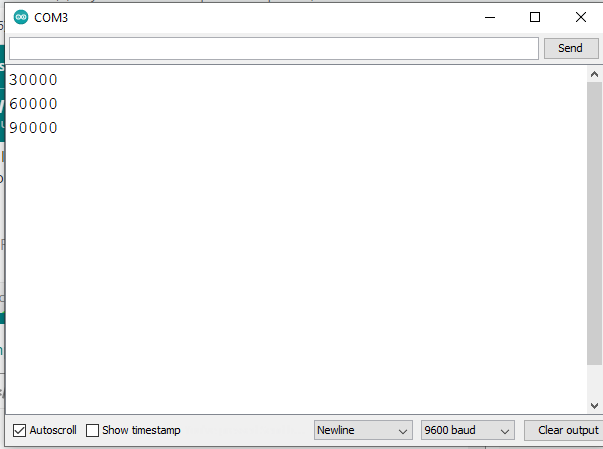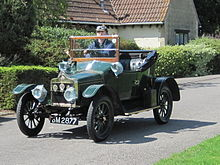# Why doesn't this simple for loop work

Hi,
I want to create an empty array of size 3 and then in a for loop add 3 element containg the integers 30000, 60000 and 90000. My code:

``````int timeStep = 30000;
int timeIntervall;
int i  = 0;

void setup() {
Serial.begin(9600);
}

void loop() {

for (int t = timeStep;i<=2;t=t+timeStep){
timeIntervall[i] = t;
i++;
}
}
``````

If I print out the values for t in each step in the for loop I get 30 000, 60 000, 90 000, which is correct. But if I print out the value of timeIntervall[i] in each step its completely wrong.

an variabe of type int on 8 bit µC can hold values +-32768

so you have to declare a variable of type long instead of int

best regards Stefan

What Arduino are you using? On 8-bit Arduinos, an 'int can't hold more than 32767 and that could be your problem. Since you don't show the code that produces the output that you don't show, it is hard to speculate more.

For values over 30 000, I would use 'unsigned long' variables.

``````unsigned long timeStep = 30000;
unsigned long  timeIntervall;

void setup()
{
Serial.begin(9600);

unsigned long t = timeStep;
for (int i = 0; i < 3; i++)
{
timeIntervall[i] = t;
t += timeStep;
}

for (int i = 0; i < 3; i++)
{
Serial.println(timeIntervall[i]);
}
}

void loop()
{
}``````

A sixteen bit "int" can hold values -32768 to +32767.

char, int, long and long long are all integer types.

So it must be a 32-bit Arduino, and why the correct values are not assigned to the array is a mystery. Please provide more info, like the model of Arduino and the full code that prints the array contents and the output you see on serial monitor (copy text from Serial Monitor and paste into your post between code tags),

Well it works fine on the RPi Pico which is 32 bit... (apart from not waiting for the USB serial to stabilize).

1. Even though the values for variables are declared/shown in decimal, they are always stored as "bits" in memory locations. Accordingly, the numbers: 30000, 60000, and 90000 would be stored as: 0x7530 (written in hex base for convenience), 0xEA60, and 0x00015F90 respectively.

Note that 0x15F90 (for 90000) is written as 0x00015F90 (32-bit) due to the fact that in Computer memory, the numbers are stored as 8-bit or 16-bit or 32-bit or 64-bit chunk; where, the required sizes are maintained by appending 0s.

2. To avoid mistakes in the selection of appropriate data type, it is peferable that the hex versions of the decimal numbers are written down on note papers.

3. As the values of Step-1 are to be stored in a 3-elemnt array, the sizes of the array elements should be the same. Accordingly, we can declare the following data type for the array, which can hold number from 0 to 4 294 967 295 (0x00000000 to 0xFFFFFFFF).

`unsigned long int timeInterval; //the data type is: "unsigned long int"`

4. The int type variable holds data of this range: -32768 to 32767 (0x8000 to 0x7FFF). Obviously, the data value 90000 would not be accomodated in an int type variable.

5. The following sketch stores/prints the data correctly (Fig-1) on Arduino UNO.

``````int timeStep = 30000;
unsigned long int timeIntervall;
int i  = 0;

void setup()
{
Serial.begin(9600);
//-------------------
unsigned long int t = timeStep;
for (i = 0; i < 3; i++)
{
timeIntervall[i] = t;
t = t + timeStep;
Serial.println(timeIntervall[i], DEC);
}
}

void loop()
{

}
``````Figure-1:

@GolamMostafa please do not set a bad example to newcomers by posting images of the serial monitor! The text should be copied and pasted between code tags. Serial Plotter output must be posted as an image, of course.

Noted down with thanks.

This is not always true. On most types of Arduino, such as Uno, Mega, Nano, which have 8-bit processors, it is true, an int is 16 bits and can hold 30000, maybe 60000 if it is unsigned int, but certainly not 90000. But for more modern types of Arduino such as Due, Zero, Nano 33, ESP8266/32, an int is 32 bits. This must be true for @scorpo because they see 30000, 60000, 90000 printed correctly and their sketch uses int, not long int.

As most of the users of this Forum are working with standard Arduinos (UNO, NANO, MEGA), the int type variable is known to them as a 16-bit memory space that can hold both positive and negative numbers of this range: 0x8000 to 0x7FFF (-32768 to 32767).

Evidence?
Or more "holy writ, and screw the minorities"?

I agree. But @scorpo 's code is using int yet can hold 90000, so @scorpo is not using a "standard" Arduino.

150 out of 150 of my pupils are using UNO and NANO.

Can you spell "parochial"?

Have limited vocabulary being a non-native.

I found, somewhere in the Arduino Forum, the definition of "Standard Arduino" as having these three Learning Kits: Arduino UNO, Arduino NANO, and Arduino MEGA.

That's like "a bloke down the pub told me..."!

There have been many types of Arduino over the years, some officially branded Arduino and others, many of them from well known and respected names like Adafruit, Sparkfun etc. I don't think there is a "Standard" Arduino any more than there is a standard raspberry pi. There was, however a Standard car, and any other car is therefore non-standardActually, "Standard" was the make and they made quite a few models, so there isn't even a standard Standard!

That's the great thing about standards - there are so many to choose.

Google translate to my language give "parish" , "community", "narrow". But I think it comes from a greek word meaning "outdated".

Pls be so kind to give the meaning of the word "parochial" .